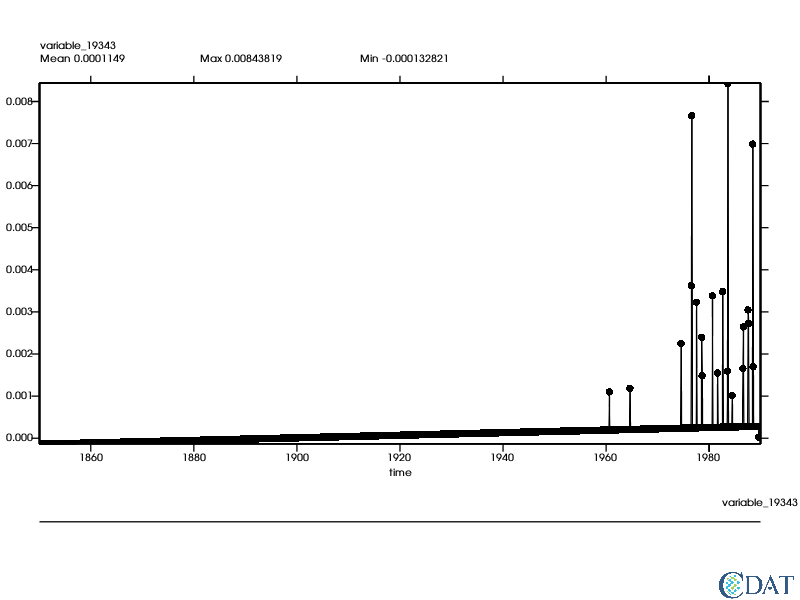# Detrend Data¶

This tutorial shows how to detrend data - a commonly-used technique prior to detailed climate data analysis - using CDAT.

The CDAT software was developed by LLNL. This tutorial was written by Charles Doutriaux. This work was performed under the auspices of the U.S. Department of Energy by Lawrence Livermore National Laboratory under Contract DE-AC52-07NA27344.

Download the Jupyter Notebook

## Prepare Notebook and Data¶

Back to Top

In :
from __future__ import print_function
import cdms2
import MV2
import genutil
import cdutil
import vcs
import os
import requests


### Download Data¶

The following NetCDF file contains Near-Surface Air Temperature data in degrees Kelvin (K) over North America. It is downloaded to the directory where this notebook is stored.

In :
filename = 'tas_Amon_IPSL-CM5A-LR_1pctCO2_r1i1p1_185001-198912.nc'
if not os.path.exists(filename):
r = requests.get("https://cdat.llnl.gov/cdat/sample_data/notebooks/{}".format(filename), stream=True)
with open(filename,"wb") as f:
for chunk in r.iter_content(chunk_size=1024):
if chunk:  # filter local_filename keep-alive new chunks
f.write(chunk)


### Open Data File, Extract Variable¶

The following two lines of code open the file just downloaded to your local computer (filename) and extract data for the Near-Surface Air Temperature (tas) and assign it to the variable data.

In :
f = cdms2.open(filename)

data = f("tas")


Let's take a quick look at the data using the .info() method. Since this file is a subset of the globe, the data cover 13 latitude bands and 16 longitude values. There are 1680 different time values which are measured as "days since 1850-01-01 00:00:00". The range of time values is the difference between the last value (51084.5) and the first value (15.5) which equals 51069 days. If we divide this range by the number of values in the dataset (51069/1680) we get an average time duration for each data point of 30.398 days which tells us that we are working with monthly data.

In :
data.info()

*** Description of Slab tas ***
id: tas
shape: (1680, 13, 16)
filename:
missing_value: 1e+20
comments:
grid_name: <None>
grid_type: generic
time_statistic:
long_name: Near-Surface Air Temperature
units: K
tileIndex: None
original_name: t2m
associated_files: baseURL: http://cmip-pcmdi.llnl.gov/CMIP5/dataLocation gridspecFile: gridspec_atmos_fx_IPSL-CM5A-LR_1pctCO2_r0i0p0.nc areacella: areacella_fx_IPSL-CM5A-LR_1pctCO2_r0i0p0.nc
coordinates: height
standard_name: air_temperature
cell_methods: time: mean (interval: 30 minutes)
cell_measures: area: areacella
history: 2011-03-07T11:45:34Z altered by CMOR: Treated scalar dimension: 'height'. 2011-03-07T11:45:34Z altered by CMOR: replaced missing value flag (9.96921e+36) with standard missing value (1e+20). 2011-03-07T11:45:34Z altered by CMOR: Inverted axis: lat.
Grid has Python id 0x112c4f5c0.
Gridtype: generic
Grid shape: (13, 16)
Order: yx
** Dimension 1 **
id: time
Designated a time axis.
units:  days since 1850-01-01 00:00:00
Length: 1680
First:  15.5
Last:   51084.5
Other axis attributes:
axis: T
calendar: noleap
realtopology: linear
long_name: time
standard_name: time
Python id:  0x12ea31240
** Dimension 2 **
id: lat
Designated a latitude axis.
units:  degrees_north
Length: 13
First:  25.578947067260742
Last:   48.31578826904297
Other axis attributes:
axis: Y
realtopology: linear
long_name: latitude
standard_name: latitude
Python id:  0x12ea31d68
** Dimension 3 **
id: lon
Designated a longitude axis.
units:  degrees_east
Length: 16
First:  -123.75
Last:   -67.5
Other axis attributes:
axis: X
modulo: 360.0
topology: circular
realtopology: circular
long_name: longitude
standard_name: longitude
Python id:  0x112c4f278
*** End of description for tas ***


### Set-up for Demonstrating That Order Matters¶

To demonstrate genutil's capabilities and highlight a scientific point about the order of operations, let's mask (delete) some data and compute the time series.

We'll delete, or mask, all data values where the maximum temperature is less 2 degrees Kelvin (K) - data.max()-2.

In :
data_masked = MV2.masked_greater(data, data.max()-2)


Next we'll create a time series of the masked data that will span the entire 1680 time values in the data file.

In :
data_masked_ts = genutil.averager(data_masked, axis='xy')


Let's take a look at the time series. Note the trend and the annual cycle.

In :
x = vcs.init(bg=True, geometry=(800,600))
line = vcs.create1d()
line.markersize = .5
x.plot(data_masked_ts, line)

Out: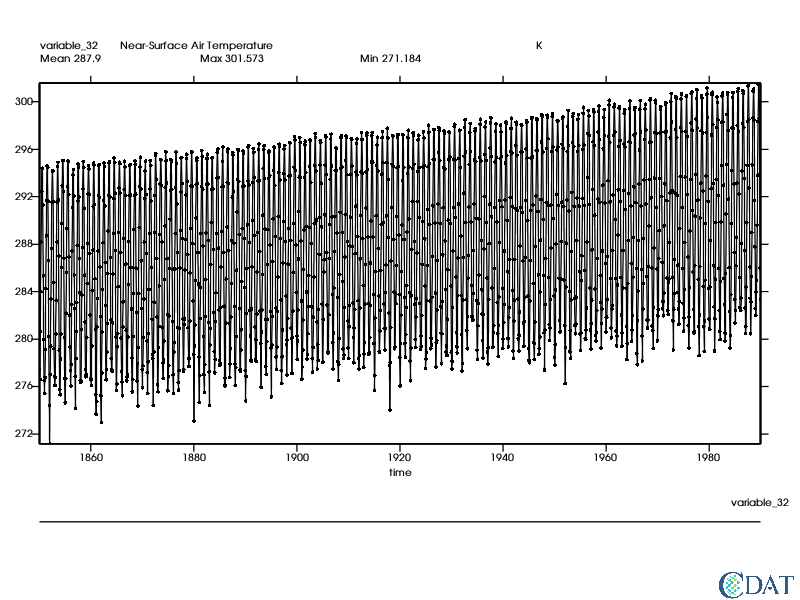## Removing the Annual Cycle¶

Back to Top

First we will remove the annual cycle for these monthly data. Because the data are masked (i.e. some data are missing) the order of operation matters, as we will demonstrate.

In :
data_departures = cdutil.times.ANNUALCYCLE.departures(data_masked) # extract the departures of the original data.
data_departures_ts = genutil.averager(data_departures, axis='xy') # create time series of the data departures.
data_ts_departures = cdutil.times.ANNUALCYCLE.departures(data_masked_ts) # extract the departures of the original data_ts time series data.


Notice how the trend shows up much nicer now!

In :
x.clear()
x.plot(data_departures_ts)

Out: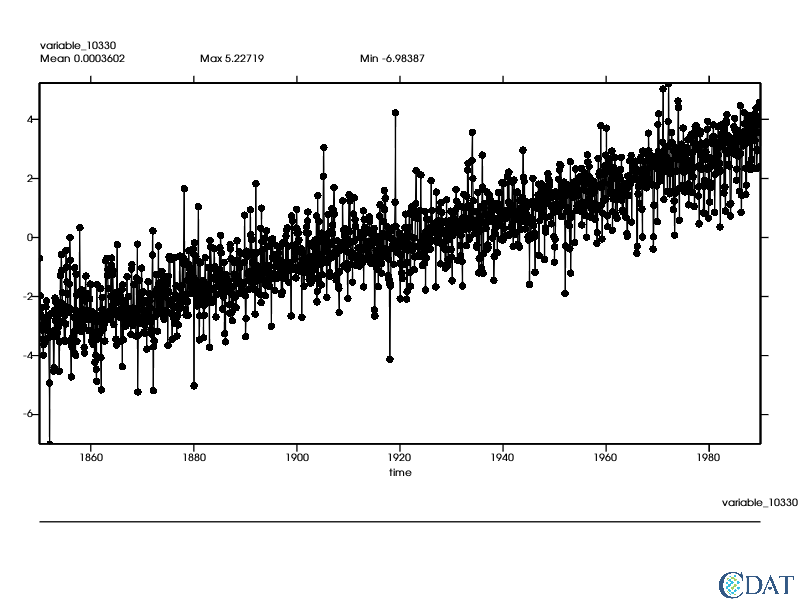Please note the importance of the order of operation when missing data is present. The two time series are slightly different especially in the later years, where missing data occurs since the temperature crosses the threshold to be removed (2 degrees cooler than the maximum temperature).

In :
x.clear()
x.plot(data_departures_ts - data_ts_departures)

Out: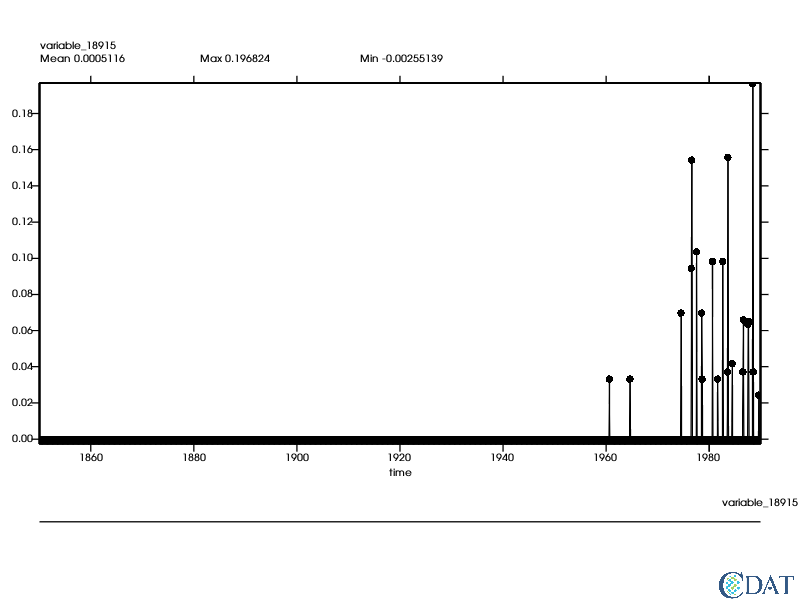## Detrend Data¶

Back to Top

First we will compute the trend over time. Note that the index of time can be anything; genutil will determine its index.

After computation we lose the time axis. Also note the units: since the time axis units are in days since XXX, the coeff/trend is in K/day.

In :
coeff, intercept = genutil.statistics.linearregression(data_departures, axis="t")
print("Shapes: coeff {}, intercept {}".format(coeff.shape, intercept.shape))
# Let's do the same for the time series
coeff_ts, intercept_ts = genutil.statistics.linearregression(data_departures_ts, axis="t")

Shapes: coeff (13, 16), intercept (13, 16)


Now let's actually remove the trend.

In :
times = MV2.array(data_masked.getTime()[:])
times.setAxis(0, data_masked.getTime())

# since time is not necessarily on index 0 we need to use the grower function
# we use data as the first argument to ensure the same order
tmp, full_times = genutil.grower(data_departures, times)
# same for cefficient
tmp, coeff = genutil.grower(data_masked, coeff)
# same for intercept
tmp, intercept = genutil.grower(data_masked, intercept)

data_departures_detrend = data_departures - full_times * coeff - intercept
data_departures_ts_detrend = data_departures_ts - times * coeff_ts - intercept_ts
x.clear()
x.plot(data_departures_ts_detrend)

Out: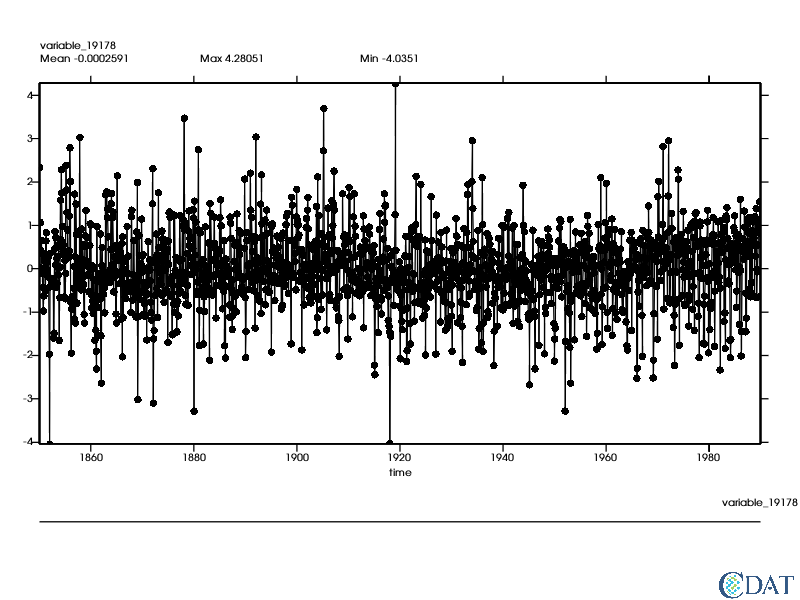Here, again, the order matters.

In :
data_departures_detrend_ts = genutil.averager(data_departures_detrend, axis='xy')

x.clear()
x.plot(data_departures_detrend_ts - data_departures_ts_detrend)

Out: